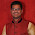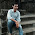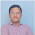Thursday, 30 April 2015

One Dimensional (1D) Image Convolution in CUDA

First let me tell you that if you are reading this page then you are already looking for some advance stuff in today's technology as both CUDA & Image Processing are highly demanding as well as advanced technologies. On this blog we will be mainly focusing on use of CUDA(Compute Unified Device Architecture) technology to improve Image Processing Algorithms.The improvement is mainly with respective to Time & Space required to Image Processing Algorithm. You may refer to concern links provided to get more information about both the fields.
Convolution is one of the most basic algorithm in Image Processing. Image convolution is required number of times as it includes various uses as Image Filtering, Image Masking, etc.
Image Convolution is again categorized as 1-Dimensional (1D) Convolution, 2-Dimensional Convolution (2-D). I would like to start this discussion with 1D Image Convolution and then we will move on to 2D Image Convolution. Now, Lets categorize the example and start knowing each part of this concept. I have categorized the concept as Input, Output & Sample Code for the sake of Implementation.
1. INPUT :
• Assume that we do have a 1D Image array with intensity values or pixel elements. Also we do have 1D Kernel of size 3 with all values of kernel as '1'.
2. OUTPUT :
• We will get output as a new Image with the new pixel values after the convolution. This new image will be of the same size as our input image.
3. SAMPLE CODE :
• We need to write the convolution code which will perform the task and produce Output for us. Here what I want to suggest is we must write a CPU function to execute on the CPU serially and after this implementation we may go for the parallelization of the same function to gain speed up. (You may say we will port the same algorithm on GPU with help of CUDA implementation).
Today instead of going into all the details of Image convolution, I am providing a sample code for the 1-D Image convolution. Please refer to following sample code.

You can also get it from my GitHub repository.

#include<stdio.h>
#include<stdlib.h>
#include<cuda.h>
#include<cuda_runtime_api.h>
#define funcCheck(stmt) do {                                                  \
cudaError_t err = stmt;                                               \
if (err != cudaSuccess) {                                             \
printf( "Failed to run stmt %d ", __LINE__);                      \
printf( "Got CUDA error ...  %s ", cudaGetErrorString(err));      \
return -1;                                                        \
}                                                                     \
} while(0)

__global__ void convolution_1D(float *N,float *M,float *P,int Mask_width,int width)
{
float Pvalue=0.0;
{
if(((N_start_point+j)>=0) && ((N_start_point+j)<width))
{
Pvalue+=N[N_start_point+j]*M[j];
}
}
P[i]=Pvalue;
}

int main()
{
float * input;
float * output;
float * device_input;
float * device_output;
int width=5;

input=(float *)malloc(sizeof(float)*width);
output=(float *)malloc(sizeof(float)*width);
for(int i=0;i<width;i++)
{
input[i]=1.0;
}
{
}

printf("\nInput:\n");
for(int i=0;i<width;i++)
{
printf("%0.2f ",input[i]);
}printf("\n");

{
}printf("\n");

funcCheck(cudaMalloc((void **)&device_input,sizeof(float)*width));
funcCheck(cudaMalloc((void **)&device_output,sizeof(float)*width));

funcCheck(cudaMemcpy(device_input,input,sizeof(float)*width,cudaMemcpyHostToDevice));

cudaError_t err1 = cudaPeekAtLastError();

printf( "Got CUDA error ... %s \n", cudaGetErrorString(err1));
funcCheck(cudaMemcpy(output,device_output,sizeof(float)*width,cudaMemcpyDeviceToHost));

printf("\n\nOutput: \n");

for(int i=0;i<width;i++)
{
printf(" %0.2f \t",*(output+i));
}

cudaFree(device_input);
cudaFree(device_output);

free(input);
free(output);

printf("\n \nNumber of Blocks Created :%d",(((width-1)/Mask_width)+1));
printf("\n \nNumber of Threads Per Block created in code: %d",(Mask_width));

return 0;
}

The expected output of the above code will be as following:
You may change the values of Input Image and Mask. I have taken all values as 1 for the simplicity of the example.

SAMPLE OUTPUT:

Input:
1.00 1.00 1.00 1.00 1.00

1.00 1.00 1.00

Output:
2.00   3.00   3.00   3.00   2.00

Number of Blocks Created :2

Number of Threads Per Block created in code: 3

Posted By ==> Yogesh Desai

You are Visitor Number:

1.Nice...useful info...

2.Thank you. Stay tunned for more of Image Processing and CUDA algorithms.

3.Good Stuff.. Liked it! :)

4.Nice sir useful information

5.Awesome article.. Very informative...!!